# User:Tohline/Appendix/Ramblings

(Difference between revisions)
 Revision as of 16:17, 16 June 2020 (view source)Tohline (Talk | contribs) (→Ramblings)← Older edit Revision as of 19:38, 6 July 2020 (view source)Tohline (Talk | contribs) (→Mathematics)Newer edit → Line 119: Line 119:
• [[User:Tohline/2DStructure/ToroidalCoordinateIntegrationLimits|Toroidal Coordinate Integration Limits]] [itex]~\Leftarrow ~~[/itex]  Includes Table of Example K(k) and E(k) Function Values
• [[User:Tohline/2DStructure/ToroidalCoordinateIntegrationLimits|Toroidal Coordinate Integration Limits]] [itex]~\Leftarrow ~~[/itex]  Includes Table of Example K(k) and E(k) Function Values
• [[User:Tohline/2DStructure/ToroidalCoordinates#Using_Toroidal_Coordinates_to_Determine_the_Gravitational_Potential|Using Toroidal Coordinates to Determine the Gravitational Potential]] (Initial Presentation)
• [[User:Tohline/2DStructure/ToroidalCoordinates#Using_Toroidal_Coordinates_to_Determine_the_Gravitational_Potential|Using Toroidal Coordinates to Determine the Gravitational Potential]] (Initial Presentation)
• +
• [[User:Tohline/2DStructure/ToroidalGreenFunction#Appendix_B:_Elliptic_Integrals|Using Toroidal Coordinates to Determine the Gravitational Potential]] (Improved Presentation)   [itex]~\Leftarrow[/itex]    includes [[User:Tohline/2DStructure/ToroidalGreenFunction#Series_Expansions|series expansions]] for K(k) and E(k)
• [[User:Tohline/Appendix/Mathematics/ToroidalFunctions|Relationships between Toroidal Functions]] [itex]~\Leftarrow ~~[/itex] 5 plots of [[[User:Tohline/Appendix/References#MF53|MF53]]] data included here
• [[User:Tohline/Appendix/Mathematics/ToroidalFunctions|Relationships between Toroidal Functions]] [itex]~\Leftarrow ~~[/itex] 5 plots of [[[User:Tohline/Appendix/References#MF53|MF53]]] data included here
• [[User:Tohline/Appendix/Mathematics/ToroidalConfusion|Confusion Regarding Whipple Formulae]]
• [[User:Tohline/Appendix/Mathematics/ToroidalConfusion|Confusion Regarding Whipple Formulae]]

## Ramblings

Sometimes I explore some ideas to a sufficient depth that it seems worthwhile for me to archive the technical derivations even if the idea itself does not immediately produce a publishable result. This page, which has a simple outline layout, provides links to these various pages of technical notes.

1. Relationship between HNM82 models and T1 coordinates
2. Orthogonal Curvilinear Coordinates
3. Playing with the Spherical Wave Equation
4. Analyzing Azimuthal Distortions
5. Integrals of Motion
1. Old discussion
2. T3 Coordinates
1. Special (quadratic) case: Joel's Derivation vs. Jay's Derivation
3. Killing Vector Approach; Jay Call's related Talk page
4. Characteristic Vector for T3 Coordinates
5. T4 Coordinates (Abandoned by Joel 7/6/2010 because non-orthogonal)
7. Photosphere of Stably Accreting DWD
8. Binary Polytropes
9. Initial Effort to Explain Jay Call's Hybrid Scheme in the Context of Zach Byerly's Dissertation
10. Exploring the Properties of Radial Oscillations in Pressure-Truncated n = 5 Polytropes
11. Instabilities Associated with Equilibrium Sequence Turning Points
12. Derivations Related to Ledoux's Variational Principle
13. More on Zero-Zero Bipolytropes
14. Analyzing Five-One Bipolytropes
15. On the Origin of Planetary Nebulae (Investigation Resulting from a July, 2013 Discussion with Kundan Kadam)</lli>
16. Looking outward, from Inside a Black Hole
17. Radial Dependence of the Strong Nuclear Force
18. Dyson (1893a) Part I:  Some Details
20. Saturn
21. Doctoral students Tohline has advised over the years
22. For Richard H. Durisen
23. Riemann Meets COLLADA and Oculus Rift S: Example (b/a, c/a) = (0.41, 0.385)
1. Virtual Reality and 3D Printing
2. Success Importing Animated Scene into Oculus Rift S
3. Carefully (Re)Build Riemann Type S Ellipsoids Inside Oculus Rift Environment
4. Other Example S-type Riemann Ellipsoids:
24. Bordeaux University

## Mathematics

1. Roots of Cubic Equation
1. PP Tori — Also includes cube root of a complex number
2. Srivastava's F-Type solution for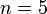$~n=5$ polytropes.
3. Murphy & Fiedler's Bipolytrope with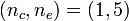$~(n_c, n_e) = (1,5)$
4. Analytic Eigenfunctions for Bipolytropes with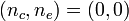$~(n_c, n_e) = (0, 0)$ — also involves cube root of a complex number
2. Roots of Quartic Equation
1. Analytic Eigenfunction for Bipolytropes with$~(n_c, n_e) = (0, 0)$
2. Determine temperature from total pressure
3. Singular Sturm-Liouville (eigenvalue) Problem
1. Oscillations of PP Tori in the slim torus limit
2. Characteristics of unstable eigenvectors in self-gravitating tori
4. Approximate Power-Series Expressions
5. Fourier Series
6. Special Functions & Other Broadly Used Representations
7. Green's Function in terms of Toroidal Functions
1. Compact Cylindrical Green Function
2. Toroidal configurations & related coordinate systems — Includes EUREKA! moment; also uses wikitable overflow (scrolling) box
3. Toroidal Coordinate Integration Limits$~\Leftarrow ~~$ Includes Table of Example K(k) and E(k) Function Values
4. Using Toroidal Coordinates to Determine the Gravitational Potential (Initial Presentation)
5. Using Toroidal Coordinates to Determine the Gravitational Potential (Improved Presentation)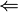$~\Leftarrow$    includes series expansions for K(k) and E(k)
6. Relationships between Toroidal Functions$~\Leftarrow ~~$ 5 plots of [MF53] data included here
7. Confusion Regarding Whipple Formulae
8. Pulling It All Together$~\Leftarrow ~~$ 2 additional plots of [MF53] data included here

## Computer-Generated Holography

 Computer Generated Holgram (Fall 2004)in collaboration with Richard Muffolettoand others from utsouthwestern.edu as cited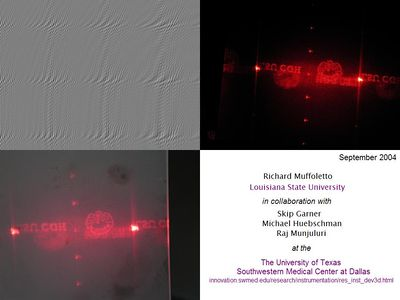2. Apertures that are Parallel to the Image Screen:
1. One-dimensional Aperture
1. Initial Ideas
2. Consolidate Expressions
3. T. Kreis, P. Aswendt, & R. Höfling (2001), Optical Engineering, vol. 40, no. 6, 926 - 933:   Hologram reconstruction using a digital micromirror device
2. Two-dimensional, Rectangular Aperture
3. Relevance to Holograms
4. Caution and Words of Wisdom
3. Apertures that are Tilted with Respect to the Image Screen:
4. Building Holograms from VRML Files:
5. ZebraImaging and Southwestern Medical Center
7. Quantum Mechanics